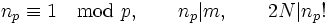# Small-index subgroup technique

This is a survey article related to:Sylow's theorem
View other survey articles about Sylow's theorem

## Description

The small-index subgroup technique basically starts off with the assumption that there is a simple non-abelian group satisfying certain conditions (most simply, having a given order$N$), and then tries to find a contradiction by finding subgroups of smaller index.

There are thus two main steps to the technique:

• Getting hold of a proper subgroup of small index.
• Using this subgroup to derive a contradiction.

## Possible contradictions from a proper subgroup of small index

### The main result: embedding in a symmetric group

The main result we use is as follows:

This states that if$H$ is a proper subgroup of$G$, and$G$ is a simple non-abelian group, then$G$ is isomorphic to a subgroup of the symmetric group$\operatorname{Sym}(G/H)$. The idea is to consider the action on the left coset space of$H$ by left multiplication, and observe that this action gives a nontrivial homomorphism from$G$ to$\operatorname{Sym}(G/H)$. Simplicity of$G$ then yields that the homomorphism is injective.

### The simplest corollary: order considerations

The most basic corollary of this result involves orders:

This states that if$H$ is a proper subgroup of a simple non-abelian group$G$, and$[G:H]$ denotes the index of$H$ in$G$, then the order of$G$ divides$[G:H]!$. This follows directly by combining the previous result with Lagrange's theorem.

This result indicates that a simple non-abelian group cannot have proper subgroups of very small index. For instance, a simple non-abelian group of order$72$ cannot have a proper subgroup of index$2,3,4,5$.

With a little more effort, we can establish the following:

In other words, if$H$ is a proper subgroup in$G$ of finite index, and$G$ is simple non-abelian, then$G$ is isomorphic to a subgroup, not just of$\operatorname{Sym}(G/H)$, but also of$\operatorname{Alt}(G/H)$.

This gives a somewhat stronger order consideration:

### Getting beyond order considerations

Once we have established that our given simple non-abelian group is isomorphic to a subgroup of an alternating group, we need to obtain a contradiction. One method of contradiction involves the use of Sylow numbers. (Note that this is slightly different from the use of Sylow numbers to obtain a subgroup of small index). Here, we may derive a contradiction in one of these, or other, ways:

• There is a prime$p$ for which the largest power of$p$ dividing the order of$G$ is the same as that dividing$\operatorname{Alt}(G/H)$, but the$p$-Sylow number of$G$ is greater than the$p$-Sylow number of$\operatorname{Alt}(G/H)$.
• There is a prime$p$ for which the largest power of$p$ dividing the order of$G$ is the same as that dividing$\operatorname{Alt}(G/H)$, but the size of the normalizer of the$p$-Sylow subgroup in$G$ is greater than the size of the normalizer in$\operatorname{Alt}(G/H)$.

## Getting hold of a proper subgroup of small index

### Using Sylow numbers: the basics

Further information: Sylow number equals index of Sylow normalizer

Let$G$ be a finite group of order$N$, and$p$ be a prime divisor of$N$. Let$n_p$ denote the$p$-Sylow number of$G$: the number of$p$-Sylow subgroups of$G$. Since all the$p$-Sylow subgroups of$G$ are conjugate, the number$n_p$ also equals the index of any Sylow normalizer: the normalizer of any$p$-Sylow subgroup. Thus, small values of$n_p$ give rise to subgroups of small index.

Specifically, if$n_p = 1$, we get a normal Sylow subgroup, contradicting the assumption that the group is simple non-Abelian. Thus,$n_p > 1$, and we get a proper subgroup of index$n_p$. Thus, by facts stated in the previous section, the simple non-Abelian group$G$ is isomorphic to a subgroup of the symmetric group of degree$n_p$. Even better,$G$ is isomorphic to a subgroup of the alternating group of degree$n_p$.

Order considerations thus put some immediate additional constraints on the Sylow numbers:

### Combining constraints on Sylow numbers

If$G$ is a simple non-abelian group of order$N$, the above facts put additional constraints on the Sylow numbers, eliminating very small values. These constraints combine with the congruence condition on Sylow numbers and the divisibility condition on Sylow numbers. In toto, we have, for any prime divisor$p$ of$N$ with$N = p^km$ for$m$ relatively prime to$p$:$n_p \equiv 1 \mod p, \qquad n_p | m, \qquad 2N | n_p!$.

### Eliminating larger possible Sylow numbers

The small-index subgroup technique eliminates very small possibilities for Sylow numbers. To eliminate the larger possibilities, we need other complementary methods. Some of these include enumeration methods: this works when$N$ has prime divisors with very small multiplicities (i.e., the largest power of the prime dividing$N$ is very small).

To eliminate small possibilities that are not very small, we need the more sophisticated techniques that use Sylow numbers in$G$ and in the alternating group it is embedded in to derive a contradiction. Some illustrative examples are done below.

## Some examples

### Elimination using the basic methods

We first discuss some examples of elimination that combine the congruence and divisibility conditions with the order constraints on Sylow numbers:

•$N = 24 = 2^3 \cdot 3$: Here, we have$n_2 \le 3$, but$24$ does not divide$3!/2$, a contradiction.
•$N = 36 = 2^2 \cdot 3^2$: Here, we have$n_3 \le 4$, but$36$ does not divide$4!/2$, a contradiction.
•$N = 48 = 2^4 \cdot 3$: Here, we have$n_2 \le 3$, but$48$ does not divide$3!/2$, a contradiction.
•$N = 72 = 2^3 \cdot 3^2$: Here, we have$n_3 | 8$ and$n_3 \equiv 1 \mod 3$, forcing$n_3 = 1,4$. But$72$ does not divide$4!/2$.